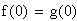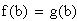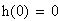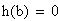Problem
At a particular horse race, two horses start at the same time, and finish in a tie. Show that at some time during the race, the horses were running at the same speed.
Solution
By carefully choosing our functions, we can solve this using Rolle's Theorem from section 2.10 in the textbook.
Let f(t) and g(t) be the distances traveled at time t by the two horses. The functions f '(t) and g'(t) are therefore the speeds of the horses at time t. Define h(t) = f(t) – g(t). Let b be the finishing time of the race. Translated into math notation, the problem states thatimplyingThus by Rolle's Theorem, there exists a time c, 0 < c < b such that h'(c) = 0. Since h(t) = f(t) – g(t), this means f '(c) – g'(c) = 0, or f '(c) = g'(c); that is, at time c the two horses are running at the same speed.